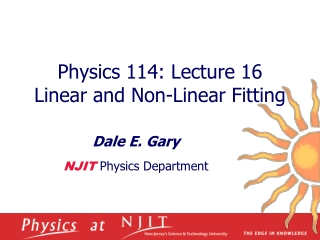Download PresentationPhysics 114: Lecture 16 Linear and Non-Linear Fitting

# Physics 114: Lecture 16 Linear and Non-Linear Fitting - PowerPoint PPT PresentationDownload Presentation## Physics 114: Lecture 16 Linear and Non-Linear Fitting

- - - - - - - - - - - - - - - - - - - - - - - - - - - E N D - - - - - - - - - - - - - - - - - - - - - - - - - - -
##### Presentation Transcript

1. Physics 114: Lecture 16 Linear and Non-Linear Fitting Dale E. Gary NJIT Physics Department

2. Reminder of Previous Results • Last time we showed that it is possible to fit a straight line of the form to any data by minimizing chi-square: • We found that we could solve for the parameters a and b that minimize the difference between the fitted line and the data (with errors si) as: where Becomes N Note, if errors si are all the same, they cancel, so can be ignored.

3. MatLAB Commands for Linear Fits • MatLAB has a “low level” routine called polyfit() that can be used to fit a linear function to a set of points (assumes all errors are equal): • x = 0:0.1:2.5; • y = randn(1,26)*0.3 + 3 + 2*x; % Makes a slightly noisy linear set of points y = 3 + 2x • p = polyfit(x,y,1) % Fits straight line and returns values of b and a in p p =2.0661 2.8803 % in this case, fit equation is y = 2.8803 + 2.0661x • Plot the points, and overplot the fit using polyval() function. • plot(x,y,'.'); • hold on • plot(x,polyval(p,x),'r') • Here is the result. The points have a scatter of s = 0.3 around the fit, as we specified above.

4. Fits with Unequal Errors • MatLAB has another routine called glmfit() (generalized linear model regression) that can be used to specify weights (unequal errors). Say the errors are proportional to square-root of y (like Poisson): • err = sqrt(y)/3; • hold off • errorbar(x,y,err,’.’) % Plots y vs. x, with error bars • Now calculate fit using glmfit() • p = glmfit(x,y) % Does same as polyfit(x,y,1) (assumes equal errors) • p = glmfit(x,y,’normal’,’weights’,err) % Allows specification of errors (weights), but must include ‘normal’ distribution type • p = circshift(p,1) % Unfortunately, glmfit returns a,b instead of b,a as polyval() wants % circshift() has the effect of swapping the order of p elements • hold on • plot(x,polyval(p,x),’r’)

5. Error Estimation • We saw that when the errors of each point in the plot are the same, they cancel from the equations for the fit parameters a and b. If we do not, in fact, know the errors, but we believe that a linear fit is a correct fit to the points, then we can use the fit itself to estimate those errors. • First, consider the residuals (deviations of the points from the fit, yi–y), calculated by • resid = y - polyval(p,x) • plot(x,resid) • You can see that this is a random distribution with zero mean, as it should be. As usual, you can calculate the variance, where now there are two fewer degrees of freedom (m = 2) because we used the data to determine a and b. • Indeed, typing std(resid) gives 0.2998, which is close to the 0.3 we used. • Once we have the fitted line, either using individual weighting or assuming uniform errors, how do we know how good the fit is? That is, what are the errors in the determination of the parameters a and b?

6. Chi-Square Probability • Recall that the value of c2 is • This should be about equal to the number of degrees of freedom, n = N – 2 = 24 in this case. Since si is a constant 0.3, we can bring it out of the summation, and calculate • sum(resid.^2)/0.3^2 ans = 24.9594 • As we mentioned last time, it is often easier to consider the reduced chi-square, which is about unity for a good fit. In this case, cn2 = 24.9594/24 = 1.04. If we look this value up in table C.4 of the text, we find that P ~ 0.4 which means if we repeated the experiment multiple times, about 40% would be expected to have a larger chi-square.

7. Uncertainties in the Parameters • We started out searching for a linear fit, , where a and b are the parameters of the fit. What are the uncertainties in these parameters? • We saw in Lecture 14 that errors due to a series of measurements propagate to the result for, e.g. a, according to • Since we have the expressions for a and b as • The partial derivatives are (note D is independent of yi)

8. Uncertainties in the Parameters • Inserting these into the expressions after some algebra (see text page 109), we have • In the case of common si = s, we have • For our example, this is calculated as • del = 26*sum(x.^2)-sum(x)^2 • siga = sum(x.^2)*0.3^2/del % gives 0.0131 • sigb = 26*0.3^2/del % gives 0.0062